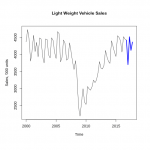Want to share your content on R-bloggers? click here if you have a blog, or here if you don't.In the previous exercises of this series, forecasts were based only on an analysis of the forecast variable. Another approach to forecasting is to use external variables, which serve as predictors. This set of exercises focuses on forecasting with the standard multivariate linear regression.
Running regressions may appear straightforward but this method of forecasting is subject to some pitfalls:
(1) a basic difficulty is selection of predictor variables (which is more of an art than a science),
(2) a possible problem is the dependence of a forecast on assumptions about expected values of predictor variables,
(3) another problem can arise if autocorrelation is present in regression residuals (it implies, among other things, that not all information, which could be used for forecasting, was retrieved from the forecast variable).
This set of exercises allow to practice in using the `regsubsets` function from the `leaps` package to run sets of regressions, making and plotting forecast from a multivariate regression, and testing residuals for autocorrelation (which requires the `lmtest` package to be installed). The model selection is based on the Bayesian information criterion (BIC).
The exercises make use of the quarterly data on light vehicles sales (in thousands of units), real disposable personal income (per capita, in chained 2009 dollars), civilian unemployment rate (in percent), and finance rate on personal loans at commercial banks (24 month loans, in percent) in the USA for 1976-2016 from FRED, the Federal Reserve Bank of St. Louis database (download here).
For other parts of the series follow the tag forecasting.
Answers to the exercises are available here.

Exercise 1
Load the dataset, and plot the `sales` variable.

Exercise 2
Create the `trend` variable (by assigning a successive number to each observation), and lagged versions of the variables `income`, `unemp`, and `rate` (lagged by one period). Add them to the dataset.
(Note that the base R libraries do not include functions for creating lags for non-time-series data, so the variables can be created manually).

Exercise 3
Run all possible linear regressions with `sales` as the dependent variable and the others as independent variables using the `regsubsets` function from the `leaps` package (pass a formula with all possible dependent variables, and the dataset as inputs to the function).
Plot the output of the function.

Exercise 4
Note that `regsubsets` returns only one “best” model (in terms of BIC) for each possible number of dependent variables. Run all regressions again, but increase the number of returned models for each size to 2.
Plot the output of the function.

Exercise 5
Look at the plots from the previous exercises and find the model with the lowest value of BIC. Run a linear regression for the model, save the result in a variable, and print its summary.

Exercise 6
Load an additional dataset with assumptions on future values of dependent variables. Use the dataset and the model obtained in the previous exercise to make a forecast for the next 4 quarters with the `forecast` function (from the package with the same name). Note that the names of the lagged variables in the assumptions data have to be identical to the names of the corresponding variables in the main dataset.
Plot the summary of the forecast.

Exercise 7
The `plot` function does not automatically draw plots for forecasts obtained from regression models with multiple predictors, but such plots can be created manually. As the first step, create a vector from the `sales` variable, and append the forecast (mean) values to this vector. Then use the `ts` function to transform the vector to a quarterly time series that starts in the first quarter of 1976.

Exercise 8
Plot the forecast in the following steps:
(1) create an empty plot for the period from the first quarter of 2000 to the fourth quarter of 2017,
(2) plot a black line for the sales time series for the period 2000-2016,
(3) plot a thick blue line for the sales time series for the fourth quarter of 2016 and all quarters of 2017.
Note that a line can be plotted using the `lines` function, and a subset of a time series can be obtained with the `window` function.

Exercise 9
Perform the Breusch-Godfrey test (the `bgtest` function from the `lmtest` package) to test the linear model obtained in the exercise 5 for autocorrelation of residuals. Set the maximum order of serial correlation to be tested to 4.
Is the autocorrelation present?
(Note that the null hypothesis of the test is the absence of autocorrelation of the specified orders).

Exercise 10
Use the `Pacf function from the forecast` package to explore autocorrelation of residuals of the linear model obtained in the exercise 5. Find at which lags partial correlation between lagged values is statistically significant at 5% level.
Residuals can be obtained from the model using the `residuals` function.